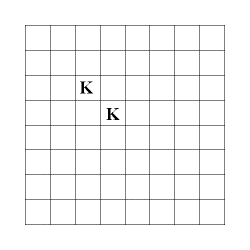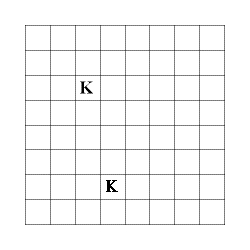Hints/clarifications on the first homework assignment.

Enumeration problems:

#1/#2 There is a north/south/east/west side to this board so don't rotate or flip it.  We are counting the number of ways that we can place two kings or two queens (indistinguishable from each other) on a chessboard so that they cannot attack each other.  Kings can move one space in any direction while queens can move any number of spaces horizontally, vertically or diagonally.

The following represents a bad placement of kings:While this is a 'good' placement#3. A partial derivative of a function is an expression of the form
d_{x_1}^{a_1} d_{x_2}^{a_2} ... d_{x_n}^{a_n} f(x_1, x_2, ..., x_n)
where each of the a_i is greater than or equal to 0.  It is a partial derivative of order r if
a_1 + a_2 + ... + a_n = r
Remember that
d_{x_i} d_{x_j} f(x_1, x_2, ..., x_n) = d_{x_j} d_{x_i} f(x_1, x_2, ..., x_n)
so partial derivatives can be taken in any order and they will be the same.
Try a few examples and you will see how this relates to combinatorics.
Here are all of the partial derivatives of f(x_1, x_2, x_3, x_4) of order 2:
d_{x_1}^2 f(x_1, x_2, x_3, x_4)
d_{x_2}^2 f(x_1, x_2, x_3, x_4)
d_{x_3}^2 f(x_1, x_2, x_3, x_4)
d_{x_4}^2 f(x_1, x_2, x_3, x_4)
d_{x_1} d_{x_2} f(x_1, x_2, x_3, x_4)
d_{x_1} d_{x_3} f(x_1, x_2, x_3, x_4)
d_{x_1} d_{x_4} f(x_1, x_2, x_3, x_4)
d_{x_2} d_{x_3} f(x_1, x_2, x_3, x_4)
d_{x_2} d_{x_4} f(x_1, x_2, x_3, x_4)
d_{x_3} d_{x_4} f(x_1, x_2, x_3, x_4)

#4.  The placing of letters in a sequence is usually a matter of choosing where the as go, choosing where the bs go, choosing where the cs go. Since there must be a b and a c between every a you might need to break this problem down into very small groups of sequences whose union is all of the sequences. Hint: There are 15 blank spaces and you need to put 5 as in them, choose instead where the 5 as will go in a sequence of 7 (21 possibilities) and then insert a b and a c after the first four.
#5.  A bridge hand has 13 cards in it.  The distribution 6-3-2-2 means that there are 6 of one suit, 3 of another suit, and 2 of each of the last two suits (e.g. 6 diamonds, 3 hearts, 2 spades and 2 clubs has a 6-3-2-2 distribution, but so does 6 hearts, 3 spades, 2 diamonds and 2 clubs).

#6. In a bridge deal each player receives 13 cards and at first you can't see the other players cards.  The players 'bid' and at the end of the bidding the person playing across from the winner lays out his/her cards so that everyone can see them.  Say that you are the winner of the bidding and the person playing across from you (your partner) puts down his/her cards and you see that between you there are 9 spades.  It is good to have some idea how many spades are in the hands of the other players.
You know what is in your hand (13 cards) and what is in your partners hand (13 cards). There are 26 other cards which you do not know, 4 of which are spades and 13 are in the hand of the player to your left and the other 13 are in the hand of the player to your right. I am asking what is the probability that either 3 of the 4 remaining cards are in the hand of the player to the left of you or that 1 of the remaining 4 cards are in the hand of the player to the left of you (because once you know the left players hand, you know the right as well).
Combinatorial proofs:
#1 There was an error on this one.  It should read:

\sum_{r=0}^n C(n,r) C(k,r) = C(n+k,n)